# Team:DLUT China/Model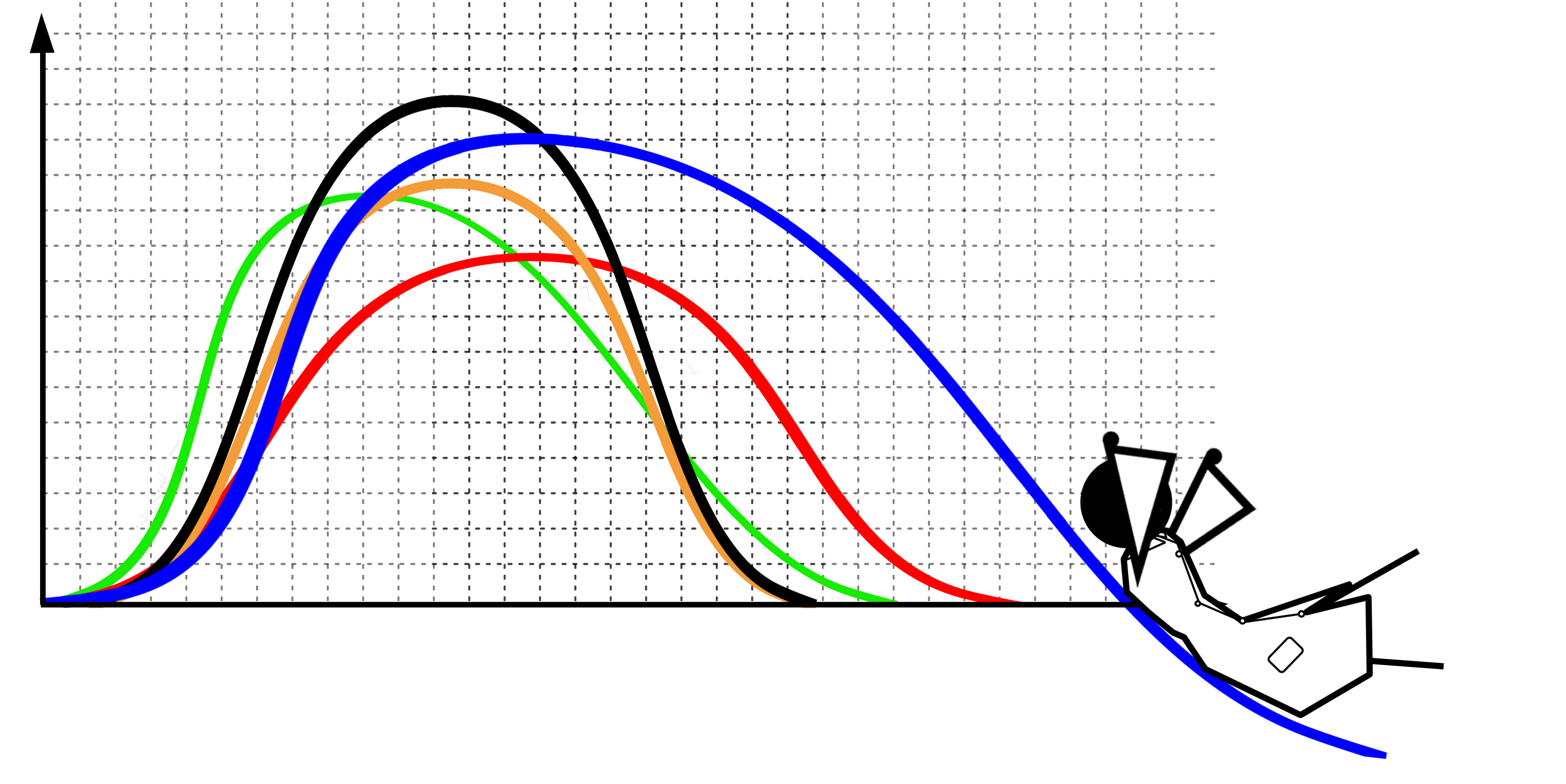# Model

I Introduction:

Our model is mainly composed of two parts: the first part is the biological part, and the first part is the Michaelis-Menten equation model, which is used to describe the relationship between the starting speed of enzymatic reaction and the substrate concentration, and then the negative feedback model of HucR-hucO-Uric Acid gene, which is used to describe the regulation of Uric Acid on the negative feedback model. The second part is a one - chamber model of the variation of uric acid oxidase concentration over time with single dose and multiple doses. According to the knowledge of pharmacology, the intensity of drug action is proportional to its concentration. When taking single and periodic drugs, we can characterize the action strength of uric acid oxidase by the concentration of uric acid oxidase in blood.
II Biological part

A The Michaelis-Menten equation model of uric acid oxidase:

In order to represent the relationship between the initial velocity of an enzymatic reaction and the substrate concentration, we chose the mistral equation to represent such a quantitative relationship

Symbol description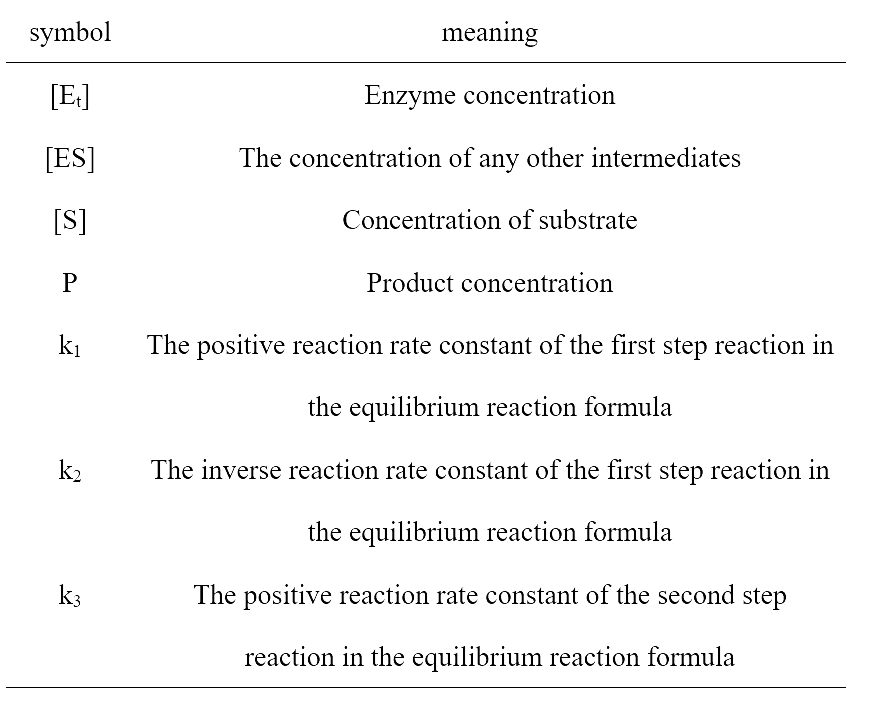First, the reaction rate and the concentration of substrate and enzyme can be obtained from the Michaelis-Menten equation.
Our chemical reaction equation can be written as:(1)
Assuming that the equilibrium of the reaction in each part of the reaction has been reached, this means that the rate of production is equal to that of the metabolic intermediates [ES] :(2)

Thus, we can infer that:(3)

We assume that :(4)

So:(5)

The reaction rate after reaching equilibrium is:(6)(7)

The relationship between the rate of enzymatic reaction and the concentration of enzyme, substrate concentration and the mil constant of enzyme is given.

B Negative feedback model of genes:

Symbol description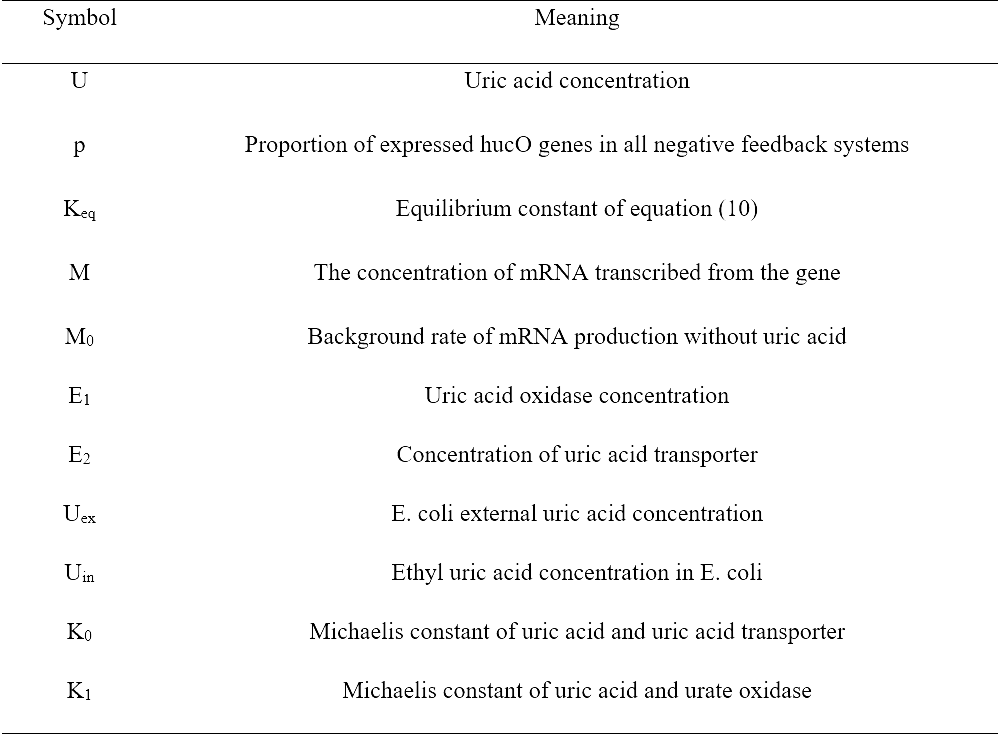HucR in the figure is a transcriptional regulatory factor, and hucR gene generates hucR protein after being expressed. Two HucR proteins can bind to form stable dimers. The dimer can interact with the promoter of flagellin and the common sequence of hucR, which is called hucO. When uric acid did not exist, the gene expressed HucR protein, forming dimer.(8)
The dimer can bind to genes,(9)
This prevents the promoter from binding to the RNA polymerase, further inhibiting the expression of related genes.
However, when uric acid is present, HucR dimer will bind to uric acid to form stable complexes, and will not bind to hucO, so that related genes can be transcribed and translated normally. Inactive genes can also bind to uric acid to restore activity.(10)
From the Griffith model, we can know: In the negative feedback model of a single gene, gene activity can be expressed by a formula(11)
In this formula, m is the number of uric acid acting on genes, which can be seen from equation (10), m=4. The activity of plasmid gene expression (proportion) was(12)
mRNA concentration (M) can change over time by the end of this rate M0, under a certain level of uric acid gene transcription rate of k1*P (P is derived from formula 11) and mRNA degradation rate k2M decided that:(13)
Where k1 and k2 are rate coefficients and both are positive values. Changes in uric acid oxidase concentration (E1) and uric acid transport protein concentration (E2) can be given by the generation and degradation of mRNA:(14)(15)
In equation (14)(15), c1,c2,d1 and d2 are rate constants, which are positive values.
The increase of uric acid concentration from extracellular to intracellular is mediated by uric acid transporter protein, while the decomposition of uric acid depends on the uric acid oxidase. As can be seen from the mistral equation (7) derived from the first part:(16)(17)
Where, σ0, σ1 are proportional coefficient, are positive.
III Plasma drug concentration model

In pharmacology, the degree of action of the drug is directly proportional to the concentration of the drug in the blood, and the efficacy of the drug can be analyzed by measuring the concentration of uric acid oxidase in the blood.
We assume that the entire human body is treated as a central chamber, a part of the body's blood. Uric acid oxidase in the central chamber is uniformly distributed and ongoing and degradation, we can set the number of e. coli enters the body, the degradation rate constant, etc., according to the function of relationship between each variable, using the extremum and differential and so on many kinds of mathematical method and the MATLAB software to solve the model, calculated the medication and periodic medication for the first time in the blood uric acid oxidase a chamber model of change over time.

Symbol description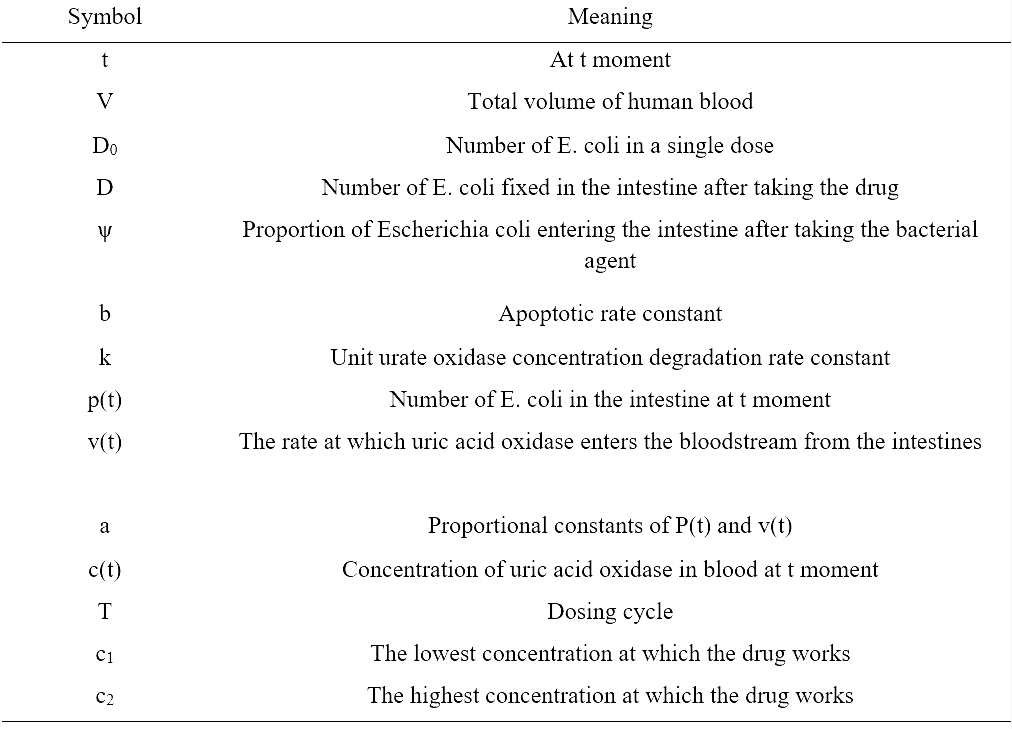A The basic assumptions:

1. The central ventricular volume, or blood volume, remains unchanged.
2. The influence of individual differences on the model is not considered.
3. Before the first entry of uric acid oxidase into the central chamber, the blood drug concentration in the central chamber was zero.
4. Treat the whole body as a central chamber, considering only the variation of uric acid oxidase concentration in blood.
5. The degradation rate of uric acid oxidase is directly proportional to the concentration of urea oxidase in blood.
6. The rate of uric acid oxidase entering blood is directly proportional to the number of escherichia coli in the intestinal tract.
7. After taking a drink or medicine, the medicine is produced and immediately enters the blood and is distributed evenly.
8. Within a reasonable range of blood drug concentration, uric acid oxidase has no side effects on the body.

B Drug distribution model

We hypothesized that the number of escherichia coli taken was D, and the number of escherichia coli taken into the human intestinal tract was p(0), then there were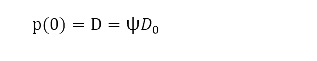(18)

After entering the intestinal tract, escherichia coli will grow, reproduce and apoptosis. It is assumed that the number of escherichia coli satisfies the differential equation with the change of time:(19)

The equation (18) and (19) satisfy the initial value problem of the differential equation: The number of coliforms in the intestinal tract at time t was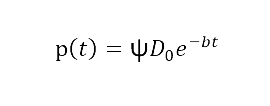(20)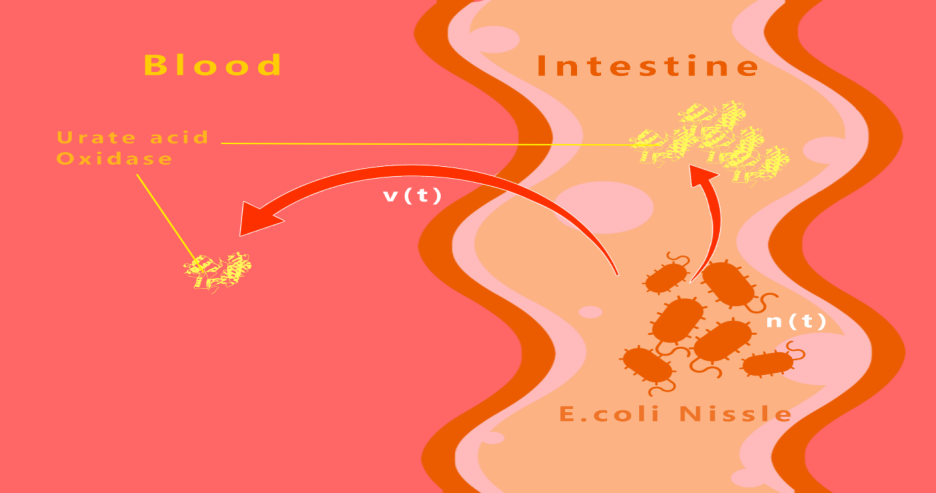##### Figure 1 transport of uric acid oxidase produced by e. coli in the intestinal tract to the blood
The rate at which uric acid oxidase enters the blood is directly proportional to its rate of production, and is directly proportional to the number of e. coli in the intestines, p(t). Therefore, the rate of uric acid oxidase entering blood v(t) is directly proportional to the number of e. coli in the intestines p(t).
The rate of uric acid oxidase entering blood from the intestinal tract can be expressed as:(21)

Where, a is the proportional coefficient of p(t) and v(t), which is a normal number.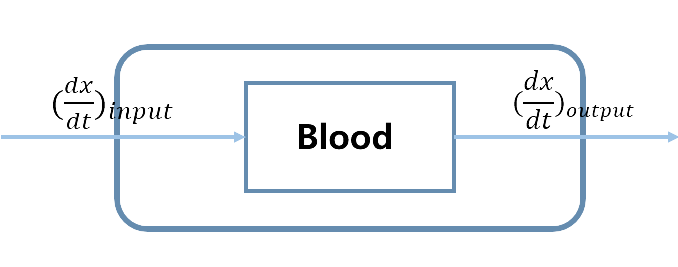##### Figure 2 Entry and degradation rate of uric acid oxidase in blood
It is assumed that the uric acid oxidase is uniformly distributed in the blood at any time, and is continuously produced and degraded. The total amount of drugs in the blood at time t is set as x(t), and the change rule of drugs in the blood satisfies the following relationship:(22)

Since the degradation rate of the drug from the blood satisfies the first-order reaction kinetics, that is, the degradation rate is proportional to the total amount of drug in the blood at the moment, and the proportionality coefficient is k, then (dx/dt)output=k*x. The rate of entering blood is (dx/dt)input=v(t). Based on the hypothesis of the above compartment model, the change of the total amount of drugs in the center at any time in the body is satisfied:(23)

Since the blood volume is, divide both sides of the equation by V, and obtain the one-room model of blood drug concentration in the center at time t:(24)

By substituting equation (21), we can get:(25)

And the first time I gave it,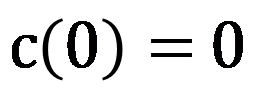(26)

So:(27)

That is, the change of uric acid oxidase concentration in blood over time, using MATLAB to draw the image as shown in figure 3.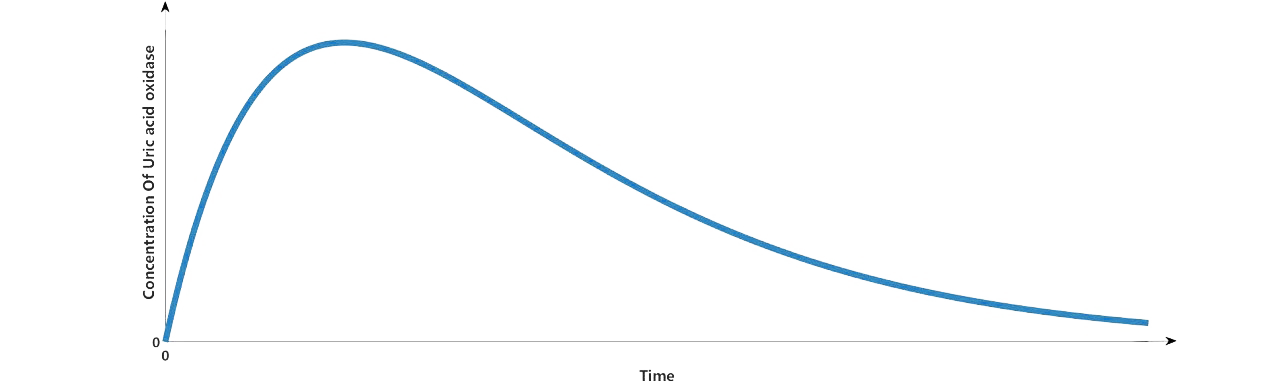##### Figure 3 c-t image of the change of uric acid oxidase concentration with time under single dose administration

Assuming that multidose function is:(28)

In the time equation of blood drug concentration after single dose administration, each index is multiplied by the multi-dose function r, where the injection cycle is set to T2, and the time equation of blood drug concentration after repeated administration is obtained(29)

When, the blood drug concentration reaches a steady state, and the relationship between the blood drug concentration and time is(30)

If I take the derivative, I get(31)

The derivative method is used to find the extremum of steady state.(32)

Thus(33)

Since cmax is no more than or equal to c2, the range of values we can obtain can be used to determine the number of escherichia coli in the bacterial agent used to guide patients to take each drug.
Combined with the single drug administration formula (27), c(T)=c1, the time interval T can be obtained under the given D0, which can be used to guide the cycle of taking the bacteria.
Finally, we make uric acid oxidase in five medication cycle concentration change over time c-t image, which, as shown, uric acid oxidase concentration in several cycle concentration is increased, but can reach the maximum concentration of uric acid oxidase less than c2, and taking inoculants, the concentration of uric acid oxidase just is equal to the lowest concentration uric acid oxidase play a role.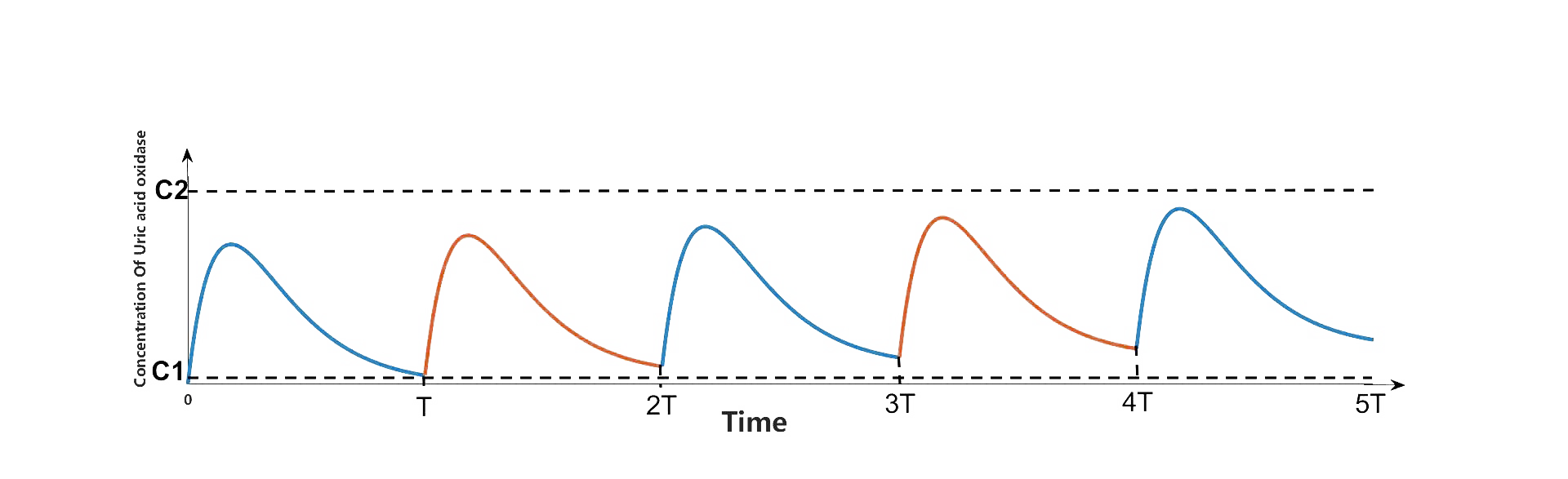##### Figure 4 c-t diagram of the change of uric acid oxidase concentration with time during cyclic administration
IV Reference

Griffith, J. (1968) Mathematics of cellular control processes I. Negative feedback to one gene. J. Theor. Biol.20 (2), 202– 208